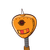# The number of students in school increase by 10%. As a result the number of teachers the school employs increases by 12 and 1/2%.

The number of students in school increase by 10%. As a result the number of teachers the school employs increases by 12 and 1/2%. The school had 120 teachers before the increase and has 1210 students after the increase. A) work out how many teachers will be employed after the increase.
B)work out how many students were in the school before the increase.

### 1 thought on “The number of students in school increase by 10%. As a result the number of teachers the school employs increases by 12 and 1/2%.”

1.the no teachers after increase=134

let the no of students before increase=x

no of students after increase =1210

no of students increased= x + 10% of x

= x + 10/100 × x

= x + x/10

ATQ

x+ x/10 = 1210

10x + x = 1210

10

11x/10 = 1210

x = 1210 × 10/11

therefore, x = 1100

so, there were 1100 students in the school before increase